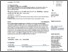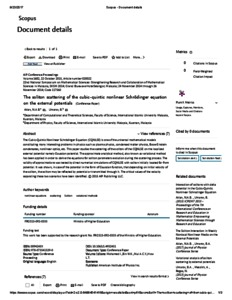The soliton scattering of the cubic-quintic nonlinear Schrödinger equation on the external potentials

Mohd Busul Aklan, Nor Amirah and Umarov, Bakhram A. (2015) The soliton scattering of the cubic-quintic nonlinear Schrödinger equation on the external potentials. AIP Conference Proceedings, 1682. 020022-1. ISSN 0094-243X (P), 1551-7616 (O)PDF - Published Version
Restricted to Repository staff onlyPreview
PDF

Abstract

The Cubic-Quintic Nonlinear Schrödinger Equation (CQNLSE) is one of the universal mathematical models constituting many interesting problems in physics such as plasma physics, condensed matter physics, Bose–Einstein condensates, nonlinear optics, etc. This paper studies the scattering of the soliton of the CQNLSE on the localized external potential namely Gaussian potential. The approximate analytical method, also known as variational method has been applied in order to derive the equations for soliton parameters evolution during the scattering process. The validity of approximations was tested by direct numerical simulations of CQNLSE with soliton initially located far from potential. It was shown, in case of the potential in the form of Gaussian function, that depending on initial velocity of the soliton, the soliton may be reflected by potential or transmitted through it. The critical values of the velocity separating these two scenarios have been identified.

Item Type: Article (Journal) 3938/45715 Soliton, nonlinear equations, scattering, variational methods Q Science > QA MathematicsQ Science > QC Physics Kulliyyah of ScienceKulliyyah of Science > Department of Physics Dr Bakhram Umarov 16 Nov 2015 09:12 29 Jan 2020 14:29 http://irep.iium.edu.my/id/eprint/45715View Item